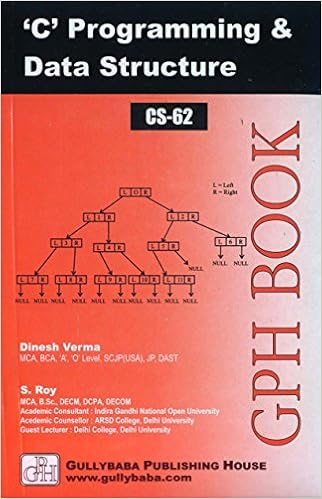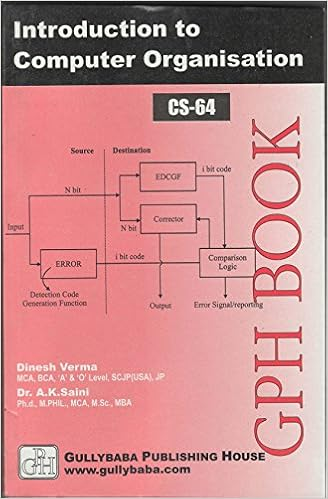### IGNOU BCA MCA.com# IGNOU Assignment mca CS-08-TMA January 2007

CS-08: Numerical and Statistical Computing
Semester: III
Assignment Type : TMA
Last date: 15th April, 2007

This is a Tutor Mark Assignment. There are three questions in this assignment. Answer all the questions. You may use illustrations and diagrams to enhance explanations. Please go through the guidelines regarding assignments.

Question 1(a): Evaluate the following FORTRAN expressions: i) 6*(7/6) + MOD(7,6). EQ.7 ii) ‘1423+’// ‘329’ iii) 24*3/4 + 18 **2**3 iv) MAX (IFIX(28–6.7), 4) v) 24 ? 5. LT. 8. OR. 7.GT.3 [marks:2]

Question 1(b): Do as directed for each of the following:
(i) Write the sequence of the numbers in which the elements of the following 3 x 3 array are stored in memory in FORTRAN environment.

 11 5 5 18 72 3 62 6 12
(ii) Find the value of the real variable x at the end of the execution of the following FORTRAN program segment: y = 3.0
Do 40 I = 1, 10, 2
Do 40 J = 1, 10, 2
y = y + 1.0
40 CONTINUE

(iii) Find the result of executing the following FORTRAN Program segment (stating clearly positions or column numbers wherever required):
I = 9
J = 12
WRITE (6, 200) I, J
200 FORMAT (‘1’, 13, 3 X, 13)
[marks:1]

Question 2(a): Solve the following system of linear equations by Gaussian elimination method, using four-digit floating point arithmetic and row interchanges:

8x + 24y ? 4z + 24 = 0
15x ? 3y + 6z ? 87 = 0
? 6x ? 8y + 2z ? 10 = 0 [marks:1]

Question 2(b): Use the Newton-Raphson method to find to three decimal places all the roots of the equation

4x3 - 4.946x2 - 21.476x + 25.526 = 0 [marks:2]

Question 3(a): The ages (x) and systolic blood pressure (y) of 12 persons are given below:

 Ages in years (x) Blood Pressure (y) 41 140 67 152 60 155 55 147 41 125 71 160 35 118 62 149 46 128 54 150 48 145 37 115
Calculate the correlation coefficient between x and y. [marks:1]

Question 3(b): Three groups of children contain 4 girls and 2 boys; 3 girls and 3 boys; and 4 boys and 2 girls. One child is selected at random from each group. Find the probability of the group of the three children so selected, consisting of 1 girl and 2 boys. [marks:1]

Here is more available MCA Assignments
 CS-02-TMA Introduction to Software, [Semester: I]MCA (1)/CS-02/TMA/20Last date: 15th April, 2007CS-02-Project Introduction to Software, [Semester: I]MCA(1)-02/Project/07Last date: 30th April, 2007CS-04-TMA Data Structures through C and Pascal, [Semester: I]MCA(1)-04/TMA/2007Last date: 15th April, 2007CS-04-Project Data Structures through C and Pascal, [Semester: I]MCA(1)-04/Project/20Last date: 30th April, 2007CS-05-TMA Elements of System Analysis and Design, [Semester: I]MCA(1)-05/TMA/2007Last date: 15th April, 2007CS-05-Project Elements of System Analysis and Design, [Semester: I]MCA(1)-05/Project/20Last date: 30th April, 2007CS-08-TMA Numerical and Statistical Computing, [Semester: III]MCA(3)-08/TMA/2007Last date: 15th April, 2007
Check other MCA Assignments